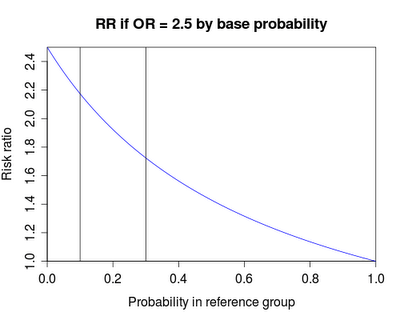Want to share your content on R-bloggers? click here if you have a blog, or here if you don't.When can you safely think of an odds ratio as being similar to a risk ratio?

Many people find odds ratios hard to interpret, and thus would prefer to have risk ratios. In response to this, you can find several papers that purport to convert an odds ratio (from a logistic regression) into a risk ratio.

Conventional wisdom has it that “odds ratios can be interpreted as risk ratios, as long as the event is rare.” Is this true? To what degree?

Let’s write a function to examine what the risk ratio is for a given odds ratio. To concretize slightly, suppose we’re examining the odds ratio and risk ratio of a “case” given the presence or absence of an “exposure.” For a given odds ratio, the risk ratio will vary depending on the baseline probability (the probability of a case in the absence of the exposure). So we’ll make the output a plot with the baseline probability as the x-axis. To aid interpretation, we’ll add vertical reference lines at baseline probabilities with default placement at 0.1 and 0.2– two values that we might think of as small enough to be able to interpret the odds ratio as a risk ratio.

SAS

In SAS, we’ll write a macro to generate the plot. First, we need to calculate the odds when the exposure is absent, then the odds when it is present (using the designated odds ratio), the probability implied by this exposed odds (for when the exposure is present), and finally the risk ratio. The coding is nothing special, with the possible exception of the loop through the base probabilities (see section 1.11.1).

```%macro orvsrr(OR=2,ref1=.1,ref2=.2);
data matt;
do p_base = 0.001, .01 to .99 by 0.01, .999;
odds_base = p_base/(1-p_base);
odds_case = &or * odds_base;
p_case = odds_case / (1 + odds_case);
RR = p_case/p_base;
output;
end;
run;

title "RR if OR = &or by base probability";
symbol1 i = j l = 1 c = blue v = none;
proc gplot data = matt;
plot RR * p_base / href = &ref1, &ref2;
label p_base = "Probability in reference group" RR = "Risk ratio";
run; quit;
%mend orvsrr;
```

The result of %orvsrr(3,.05,.1); is shown above.

R

In R, the function to replicate this is remarkably similar. In fact, the calculation of the odds, probabilities, and risk ratio is identical with the SAS version. The c() and seq functions replace the do loop used in SAS.

```orvsrr = function (or=2, ref1=.1, ref2=.2) {
p_base = c(.001, seq(.01,.99, by = .01), .999)
odds_base = p_base/(1-p_base)
odds_case = or * odds_base
p_case = odds_case / (1 + odds_case)
RR = p_case/p_base

plot(p_base, RR, xlim=c(0, 1), ylim=c(1, or), xaxs="i", yaxs="i",
xlab="Probability in reference group", ylab="Risk ratio", type = "n")
title(main = paste("RR if OR =", or, "by base probability"))
lines(p_base, RR, col="blue")
abline(v=c(ref1, ref2))
}
```

The result of orvsrr(2.5,.1,.3) is shown below.

The conventional wisdom is correct, up to a point, as you can find by playing with the function or the macro. If the baseline probability is very low (less than 0.05) and the odds ratio is smallish (less than 3 or so) then the odds ratio overestimates the risk ratio by 10% or less. Larger odds ratios or baseline probabilities result in greater overestimation of the risk ratio.